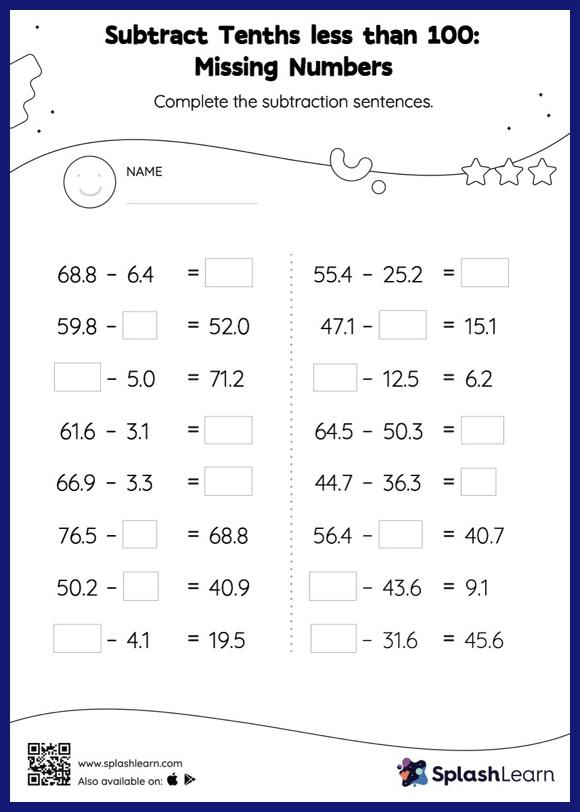# Subtract Tenths less than 100: Missing Numbers Worksheet

Home > Subtract Tenths less than 100: Missing NumbersTask your little mathematicians to crack the code of subtracting tenths less than 100 with this fun worksheet. In this worksheet, students subtract decimals by aligning the decimal points and using zero as a placeholder. They then use the relationship between addition and subtraction to find the missing number in the subtract tenths less than 100 worksheet.